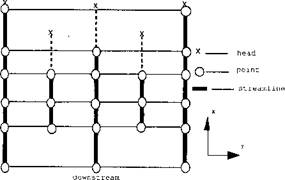## Data Structures Flow lines

The computation of microstructures at a spatial point requires that the thermal history be deduced from a set of elements or nodes in a steady state temperature field. The correct history of a material point at a downstream spatial point can be revealed only by following a flow line from its upstream origin to its downstream destination. For this reason, the flow line collection is organized as described below, and as shown in Figure 4-5.Figure 4-5: The structure of flow lines in a two-dimensional cross-section of a three-dimensional FEM mesh.

(1) Each flow line has three parts: head, body, and front end condition. The head is initial position. Front end condition is initial condition. The body is the flow line path described by a collection of points, ordered from upstream to downstream. Every point Xi with coordinates in the body stores a pointer to the

point, X^i (xM, _yM, zM ), immediately preceding it. The time increment At is easily calculated, since the velocity v is known. In this context, v is the weld speed, the weld path is assumed to be along the x-axis, and v and xj are scalars.

. Ax Дх. — Ax. ,

At = - = -^-------------------------------------- (4-22)

v v

The front end condition can be either defined from the problem data, if the flow line starts at a surface of the domain, or interpolated from the computed data within the element in which the flow line starts.

(2) The flow lines are kept in a global collection, sorted by the x - coordinate of their head positions. The computation loops over the flow lines in the sorted order, proceeding downstream along each flow line. This structural order guarantees the computing order that is necessary when a given point “p ” represents the start condition of a flow line. Only after the values of all the points, contained in an upstream element of the point llp ” have been computed can a value at “p ” be computed.

To satisfy the requirements in building the above collection of flow lines, Gu et al  created a multi-layered tree structure as described later.

Комментарии закрыты.